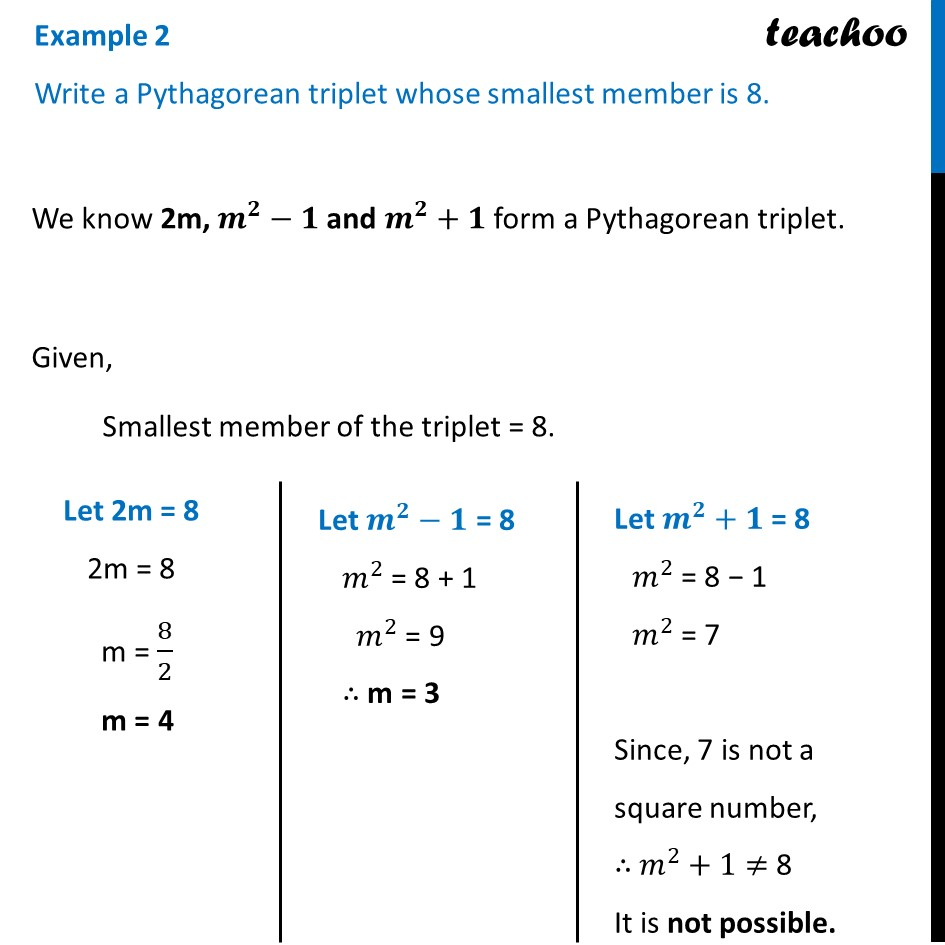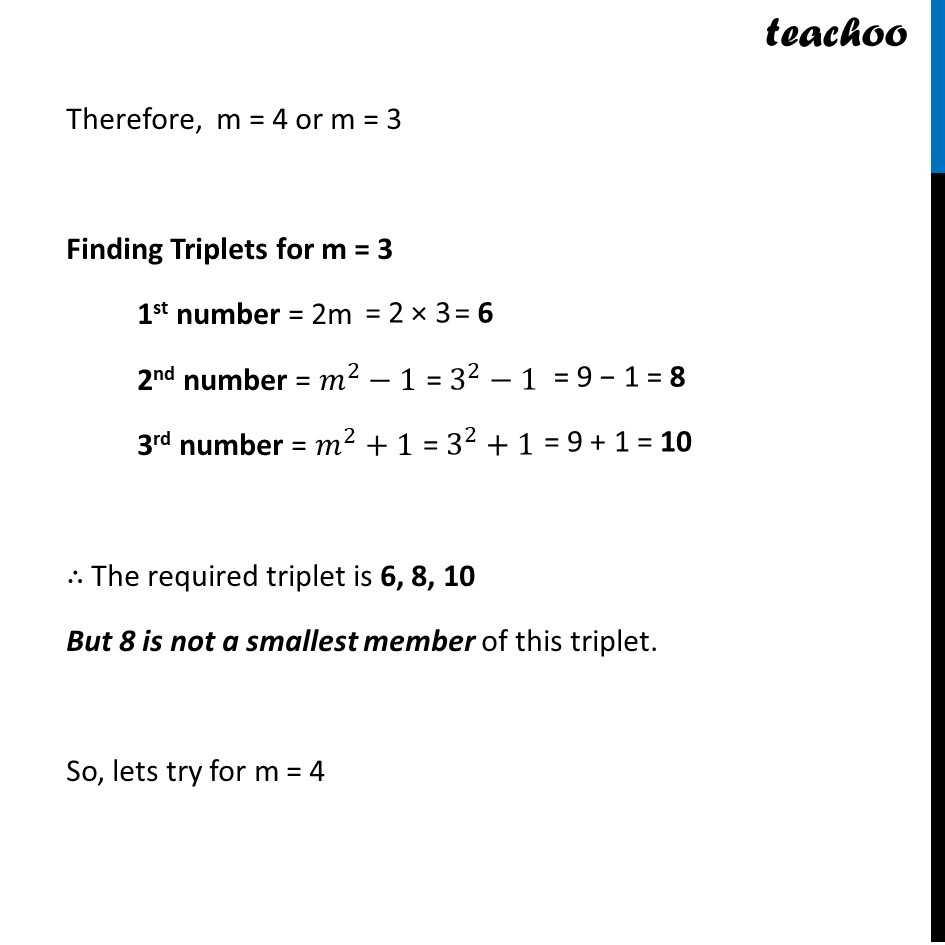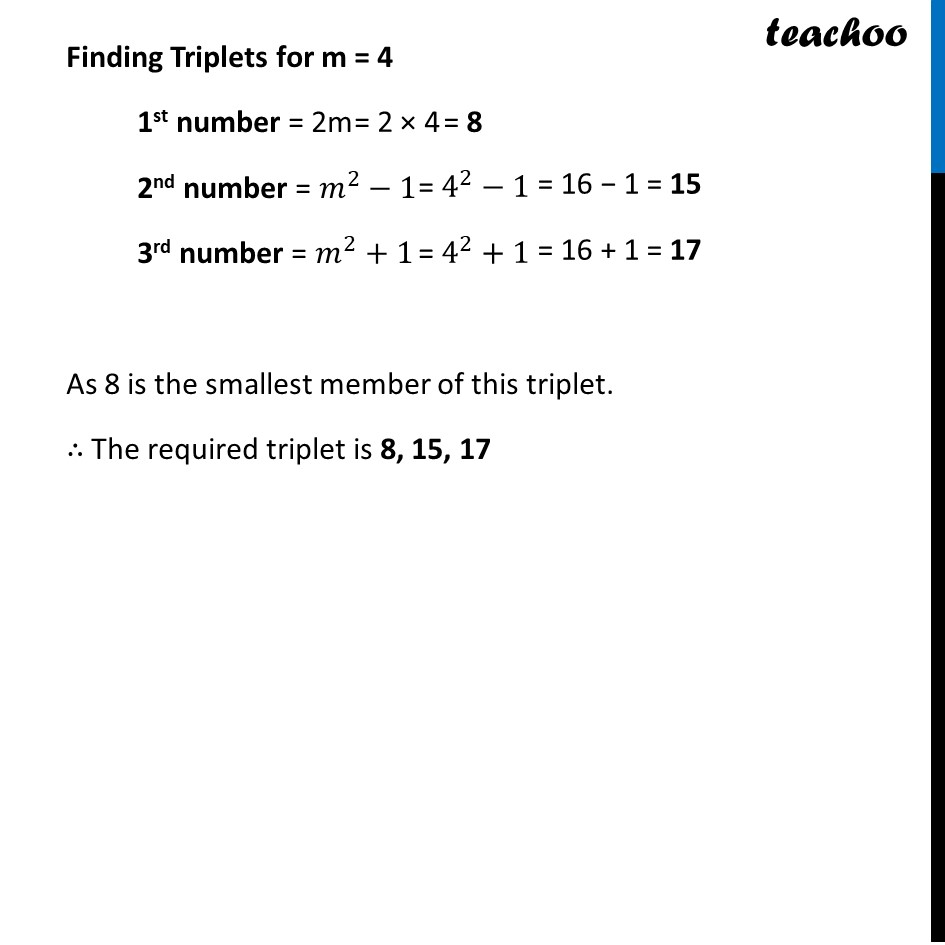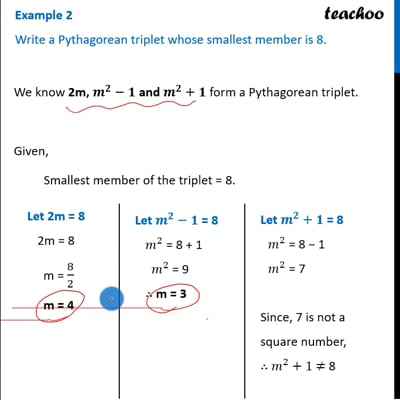Examples

Chapter 6 Class 8 Squares and Square Roots
Serial order wiseThis video is only available for Teachoo black users

### Transcript

Example 2 Write a Pythagorean triplet whose smallest member is 8. We know 2m, 𝑚^2−1 and 𝑚^2+1 form a Pythagorean triplet. We know 2m, 𝒎^𝟐−𝟏 and 𝒎^𝟐+𝟏 form a Pythagorean triplet. Given, Smallest member of the triplet = 8. Let 2m = 8 2m = 8 m = 8/2 m = 4 Let 𝒎^𝟐−𝟏" = 8" 𝑚^2 = 8 + 1 𝑚^2 = 9 ∴ m = 3 Let 𝒎^𝟐+𝟏 = 8 𝑚^2 = 8 − 1 𝑚^2 = 7 Since, 7 is not a square number, ∴ 𝑚^2+1 ≠ 8 It is not possible. Therefore, m = 4 or m = 3 Finding Triplets for m = 3 1st number = 2m 2nd number = 𝑚^2−1 3rd number = 𝑚^2+1 ∴ The required triplet is 6, 8, 10 But 8 is not a smallest member of this triplet. So, lets try for m = 4 Finding Triplets for m = 4 1st number = 2m 2nd number = 𝑚^2−1 3rd number = 𝑚^2+1 As 8 is the smallest member of this triplet. ∴ The required triplet is 8, 15, 17